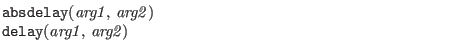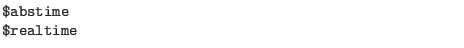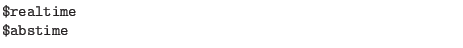Next: WRspice C/C++ Bridge Function Up: System Tasks Previous: Random Numbers   Contents   Index

#### Other System Functions Recognized in ADMS/WRspiceRecognized but not implemented.Returns the simulation time, the names are equivalent.

analysis(keyword)
Return nonzero if the analysis type represented by the keyword is being performed. The keyword is one of:
ac
True when running AC analysis.

dc
True when running DC sweep or operating point analysis.

noise
True when running noise analysis.

tran
True when running transient analysis.

ic
True in the initial-condition analysis that preceeds a transient analysis.

static
Any equilibrium point calculation, including a DC analysis as well as those that precede another analysis, such as the DC analysis that precedes an AC or noise analysis, or the IC analysis that precedes a transient analysis.

nodeset
The phase during an equilibrium point calculation where node voltages are forced.

ceil(x)
Return the integer value greater than or equal to the argument.

ddt(expression[, ignored])
Return the time derivative, any second argument is ignored.

ddx(variable, probe)
Return the partial derivative of the variable with respect to the probe.

flicker_noise(a, b[, c])
Probably not implemented.

floor(x)
Return the integer value less than or equal to the argument.

$given(model_or_instance_parameter) Return nonzero if the parameter was given, same as$param_given.

idt(expression, icval, reset[, ignored)
Return the time integral of expression using the initial value icval. If reset is nonzero, instead zero the internal integration history.

$mfactor Return the device M scale factor if the model was built for this support, otherwise 1.0.$model
Expands to the name of the current device model.

$nominal_temperature Return the nominal temperature in Kelvin.$instance
Expands to the name of the currenly scoped instance, or ???''.

$param_given(model_or_instance_parameter) Return nonzero if the parameter was given, same as$given.

$port_connected(port_name) Return nonzero if the named port is connected externally.Return the simulation time, the names are equivalent.$scale
Return 1.0, no scaling in WRspice.

$simparam(string[, expression]) This queries the simulator for a simulation parameter named in string. If string is known, its value is returned. If string is not known, and the optional expression is not supplied, then an error is generated. If the optional expression is supplied, its value is returned if string is not known and no error is generated.$simparam() shall always return a real value; simulation parameters that have integer values shall be coerced to real. There is no fixed list of simulation parameters. However, simulators shall accept the strings below to access commonly-known simulation parameters, if they support the parameter. Simulators can also accept other strings to access the same parameters.

The first group below comes from the Verilog-AMS specification.

gdev
Additional conductance to be added to nonlinear branches for conductance homotopy convergence algorithm. Returns the WRspice gmin parameter.

gmin
Minimum conductance placed in parallel with nonlinear branches, returns the WRspice gmin parameter.

imax
Branch current threshold above which the constitutive relation of a nonlinear branch should be linearized. Returns 1.0.

imelt
Branch current threshold indicating device failure. Returns 1.0.

iteration
Iteration number of the analog solver, returns an internal iteration count.

scale
Scale factor for device instance geometry parameters. Returns 1.0.

shrink
Optical linear shrink factor. Returns 1.0.

simulatorSubversion
The simulator sub-version. Returns, e.g., 5 for WRspice-4.3.5.

simulatorVersion
The simulator version. Retursn, e.g., 4.3 for WRspice-4.3.5.

sourceScaleFactor Multiplicative factor for independent sources for source stepping homotopy convergence algorithm. WRspice returns the scaling value from source stepping.

tnom
Default value of temperature in Celsius at which model parameters were extracted (same as $nominal_temperature>). The following group has unknown origin. checkjcap Returns 1.0. maxmosl Returns 1.0. maxmosw Returns 1.0. minmosl Returns 1.0e-12. minmosw Returns 1.0e-12. The final group is implemented in WRspice, perhaps uniquely. tstep The current transient analysis output time increment. tstart The current start time for transient analysis output. tstop The current final time point in transient analysis. delta The current internal time step in transient analysis. delmin The minimum allowable transient analysis time step. delmax The maximum allowable transient analysis time step. abstol The absolute tolerance parameter. reltol The relative tolerance parameter. chgtol The charge tolerance parameter. vntol The voltage tolerance parameter. predictor Nonzero when in the first iteration of a time point. smallsig Nonzero when loading small-signal values in AC analysis. dcphasemode Nonzero if using phase-mode DC analysis, may be true when Josephson junctions are present. dphimax Returns the maximum phase change for internal time point for sinusoidal sources and Josephson junctions.$temperature
Returns the circuit ambient temperature in Kelvin.

\$vt[(temperature_expression)]
Returns the thermal voltage KT/q using the argument for temerature, or the ambient temperature if no argument is given.Next: WRspice C/C++ Bridge Function Up: System Tasks Previous: Random Numbers   Contents   Index
Stephen R. Whiteley 2022-09-18